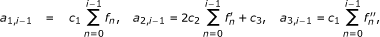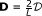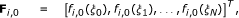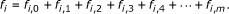# An improved spectral homotopy analysis method for solving boundary layer problems

## Abstract

This article presents an improved spectral-homotopy analysis method (ISHAM) for solving nonlinear differential equations. The implementation of this new technique is shown by solving the Falkner-Skan and magnetohydrodynamic boundary layer problems. The results obtained are compared to numerical solutions in the literature and MATLAB's bvp4c solver. The results show that the ISHAM converges faster and gives accurate results.

## Introduction

Boundary layer flow problems have wide applications in fluid mechanics. In this article, we propose an improved spectral-homotopy analysis method (ISHAM) for solving general boundary layer problems. Three boundary layer problems are considered and solved in this study using the novel technique. The first problem considered is the classical two-point nonlinear boundary value Blasius problem which models viscous fluid flow over a semi-infinite flat plate. Although solutions for this problem had been obtained as far back as 1908 by Blasius , the problem is still of great interest to many researchers as can be seen from the several recent studies .

The second problem considered in this article is the third-order nonlinear Falkner-Skan equation. The Falkner-Skan boundary layer equation has been studied by several researchers from as early as 1931 . More recent studies of the solutions of the The Falkner-Skan equation include those of Harries et al. , Pade  and Pantokratoras . The third problem considered is magnetohy-drodynamic (MHD) boundary layer flow. Such boundary layer problems arise in the study of the flow of electrically conducting fluids such as liquid metal. Owing to its many applications such as power generators, flow meters, and the cooling of reactors, MHD flow has been studied by many researchers, for example [10, 11].

Owing to the nonlinearity of equations that describe most engineering and science phenomena, many authors traditionally resort to numerical methods such as finite difference methods , Runge-Kutta methods , finite element methods  and spectral methods  to solve the governing equations. However, in recent years, several analytical or semi-analytical methods have been proposed and used to find solutions to most nonlinear equations. These methods include the Adomian decomposition method , differential transform method , variational iteration method , homotopy analysis method (HAM) , and the spectral-homotopy analysis (SHAM) (see Motsa et al. [24, 25]) which sought to remove some of the perceived limitations of the HAM. More recently, successive linearization method , has been used successfully to solve nonlinear equations that govern the flow of fluids in bounded domains.

In this article, boundary layer equations are solved using the ISHAM. The ISHAM is a modified version of the SHAM [24, 25]. One strength of the SHAM is that it removes restrictions of the HAM such as the requirement for the solution to conform to the so-called rule of solution expression and the rule of coefficient ergodicity. Also, the SHAM inherits the strengths of the HAM, for example, it does not depend on the existence of a small parameter in the equation to be solved, it avoids discretization, and the solution obtained is in terms of an auxiliary parameter ħ which can conveniently be chosen to determine the convergence rate of the solution.

## Mathematical formulation

We consider the general nonlinear third-order boundary value problem(2.1)

subject to the boundary conditions(2.2)

where c i , b j (i = 1, ..., 4 j = 1, 2, 3) are constants.

Equation 2.1 can be solved easily using methods such as the HAM and the SHAM. In each of these methods, an initial approximation f0(η) is sought, which satisfies the boundary conditions. The speed of convergence of the method depends on whether f0(η) is a good approximation of f (η) or not. The approach proposed here seeks to find an optimal initial approximation f0 that would lead to faster convergence of the method to the true solution. We thus first seek to improve the initial approximation that is used later in the SHAM to solve the governing nonlinear equation.

We assume that the solution f(η) may be expanded as an infinite sum:(2.3)

where f i 's are unknown functions whose solutions are obtained using the SHAM at the i th iteration and f n , (n ≥ 1) are known from previous iterations. The algorithm starts with the initial approximation f0(η) which is chosen to satisfy the boundary conditions (2.2). An appropriate initial guess is(2.4)

Substituting (2.3) in the governing equation (2.1-2.2) gives(2.5)

subject to the boundary conditions(2.6)

where the coefficient parameters ak,i-1, (k = 1, ..., 3) and ri-1are defined as(2.7)(2.8)

Starting from the initial approximation (2.4), the subsequent solutions f i (i ≥ 1) are obtained by recursively solving Equation 2.5 using the SHAM, [24, 25]. To find the solutions of Equation 2.5, we begin by defining the following linear operator:(2.9)

where q 0 is the embedding parameter, and F i (η; q) is an unknown function.

The zeroth-order deformation equation is given by(2.10)

where ħ is the non-zero convergence controlling auxiliary parameter andis a nonlinear operator given by(2.11)

Differentiating (2.10) m times with respect to q and then setting q = 0, and finally dividing the resulting equations by m! yield the m th-order deformation equations:(2.12)

subject to the boundary conditions(2.13)

where(2.14)

The initial approximation fi,0that is used in the higher-order equations (2.12) is obtained on solving the linear part of Equation 2.5 which is given by(2.15)

subject to the boundary conditions:(2.16)

Since the coefficient parameters and the right-hand side of Equation 2.15 for i = 1, 2, 3, ... are known (from previous iterations), the equation can easily be solved using numerical methods such as finite differences, finite elements, Runge-Kutta-based shooting methods or collocation methods. In this article, Equation 2.15 are solved using the Chebyshev spectral collocation method. The method (see, for example, ), is based on the Chebyshev polynomials defined on the interval [-1, 1] by(2.17)

To implement the method, the physical region [0, ∞) is transformed into the region [-1, 1] using the domain truncation technique whereby the problem is solved in the interval [0, L] instead of [0, ∞). This leads to the mapping(2.18)

where L is the scaling parameter used to invoke the boundary condition at infinity. We use the popular Gauss-Lobatto collocation points [29, 31] to define the Chebyshev nodes in [-1, 1], namely:(2.19)

where N is the number of collocation points. The variable fi,0is approximated by the interpolating polynomial in terms of its values at each of the collocation points by employing the truncated Chebyshev series of the form:(2.20)

where T k is the k th Chebyshev polynomial. Derivatives of the variables at the collocation points may be represented by(2.21)

where s is the order of differentiation and, withbeing the Chebyshev spectral differentiation matrix (see, for example [29, 31]) whose entries are defined as(2.22)

Substituting Equations 2.20-2.21 in 2.15-2.16 gives(2.23)

subject to(2.24)

where(2.25)(2.26)(2.27)

In the above definitions, T stands for transpose and ak,i-1(k = 1, 2, 3) denotes a diagonal matrix of size (N + 1) × (N + 1). The boundary condition f i (ξ N ) = 0 is implemented by deleting last row and last column of Ai-1, and deleting the last rows of Fi,0and Ri-1. The derivative boundary conditions in (2.24) are then imposed on the resulting first row and last row of Ai-1and setting the first and last rows of Fi,0and Ri-1to be zero. The solutions for fi.0(ξ) are then obtained from soloving(2.28)

In a similar manner, applying the Chebyshev spectral transformation on the higher order deformation equations (2.12)-(2.13) gives(2.29)

subject to the boundary conditions(2.30)

where Ai-1and Ri-1, are as defined in (2.25) and (2.27), respectively, and(2.31)(2.32)

To implement the boundary condition fi,m(ξ N ) = 0, we delete the last rows of Pi,m-1and Ri-1and delete the last row and the last column of Ai-1in (2.29). The other boundary conditions in (2.30) are imposed on the first and the last rows of the modified Ai-1matrix on the left side of the equal sign in (2.29). The first and the last rows of the modified Ai-1matrix on the right side of the equal sign in (2.29) are then set to be zero. This results in the following recursive formula for m ≥ 1:(2.33)

where Ãi-1is the modified matrix Ai-1after incorporating the boundary conditions (2.30). Thus, starting from the initial approximation, which is obtained from (2.28), higher-order approximations fi,m(ξ) for m ≥ 1, can be obtained through the recursive formula (2.33).

The solutions for f i are then generated using the solutions for fi, mas follows:(2.34)

The [i, m] approximate solution for f (η) is then obtained by substituting f i (obtained from 2.34) in equation 2.3.

## Results and discussion

Table 1 shows the values of f" (0) at different orders [i, m] of the ISHAM approximation for the Blasius boundary layer flow when L = 30, ħ = -1 and N = 80. It is worth noting here that the numerical solution given by Howarth  is f" (0) = 0.332057, while the numerical result by the Matlab bvp4c routine is f" (0) = 0.33205734. Asaithambi  found this number correct to nine decimal positions as 0.332057336. It is evident that the ISHAM converges to the numerical result at orders [3,1] and [2,2]. Moreover, Table 1 shows that the ISHAM solution converges to the accurate solution of Howarth and the bvp4c result faster than the original SHAM results of which are those given in the first row of Table 1 (for the case when i = 1).

In general, at order [i, m], i is the number of improvements of the initial approximation f0(η) for f(η), and m is the number of improvements of the initial guess f q ,0(η); q = 1, 2, ..., i, for each application of the ISHAM. Table 2 gives a sense of the convergence rate of the ISHAM when compared with the numerical method for the Blasius problem at different values of η. In all the instances, convergence of the ISHAM is achieved at the second order.

Table 3 gives the values of f" (0) obtained used the ISHAM and the numerical method for various values of β for the Falkner-Skan boundary layer problem. Full convergence is again achieved at order [2,2] for all the parameter values.

For the MHD boundary layer problem, Tables 4 and 5 illustrate the exact and approximate values of f' (η) and f" (0) at different values of η and the magnetic parameter M, respectively. The absolute errors in the approximations are also given. The tables show that the ISHAM converges rapidly with marginal or no errors after order [2,2].

## Conclusion

In this article, we have proposed an ISHAM for solving general nonlinear differential equations. This novel technique was compared against both numerical approximations and the MATLAB bvp4c routine for solving Falkner-Skan and MHD boundary layer problems. The results demonstrate the relatively more rapid convergence of the ISHAM, and they show that the ISHAM is highly accurate.

## Abbreviations

HAM:

homotopy analysis method

ISHAM:

improved spectral-homotopy analysis method

MHD:

magnetohydrodynamic

SHAM:

spectral-homotopy analysis.

## References

1. Blasius H: Grenzschichten in Flussigkeiten mit kleiner Reibung. Z Math Phys 1908, 56: 1-37.

2. Ahmad F, Al-Barakati WH: An analytic solution of the Blasius problem. Commun Nonlinear Sci Numer Simul 2009, 14: 1020-1024.

3. Alizadeh-Pahlavan A, Borjian-Boroujeni S: On the analytic solution of viscous fluid flow past a flat plate. Phys Lett A 2008, 372: 3678-3682. 10.1016/j.physleta.2008.02.050

4. Parand K, Shahini M, Dehghan M: Solution of a laminar boundary layer flow via a numerical method. Commun Nonlinear Sci Numer Simulat 2010, 15: 360-367. 10.1016/j.cnsns.2009.04.007

5. Yun BI: Intuitive approach to the approximate analytical solution for the Blasius problem. Appl Math Comput 2009, 208: 156-164. 10.1016/j.amc.2008.11.028

6. Falkner VM, Skan SW: Some approximate solutions of the boundary layer equations. Philos Mag 1931, 12: 865-896.

7. Harris SD, Ingham DB, Pop I: Unsteady heat transfer in impulsive Falkner-Skan flows: constant wall temperature case. Eur J Mech B 2002, 21: 447-468. 10.1016/S0997-7546(02)01193-7

8. Padé O: On the solution of Falkner-Skan equations. J Math Anal Appl 2003, 285: 264-274. 10.1016/S0022-247X(03)00402-5

9. Pantokratoras A: The Falkner-Skan flow with constant wall temperature and variable viscosity. Int J Thermal Sci 2006, 45: 378-389. 10.1016/j.ijthermalsci.2005.06.004

10. Rashidi MM: The modified differential transform method for solving MHD boundary-layer equations. Comput Phys Commun 2009, 180: 2210-2217. 10.1016/j.cpc.2009.06.029

11. Parand K, Rezai AR, Ghaderi SM: An approximate solution of the MHD Falkner-Skan flow by Hermite functions pseudospectral method. Commun Nonlinear Sci Numer Simulat 2010.

12. Asaithambi A: A second-order finite-difference method for the Falkner-Skan equation. Appl Math Comput 2004, 156: 779-786. 10.1016/j.amc.2003.06.020

13. Cortell R: Numerical solutions of the classical Blasius flat-plate problem. Appl Math Comput 2005, 170: 706-710. 10.1016/j.amc.2004.12.037

14. Asaithambi A: Numerical solution of the Falkner-Skan equation using piecewise linear functions. Appl Math Comput 2004, 159: 267-273. 10.1016/j.amc.2003.10.047

15. Elgazery NS: Numerical solution for the Falkner-Skan equation. Chaos Soliton Fract 2008, 35: 738-746. 10.1016/j.chaos.2006.05.040

16. Wang L: A new algorithm for solving classical Blasius equation. Appl Math Comput 2004, 157: 1-9. 10.1016/j.amc.2003.06.011

17. Abbasbandy S: A numerical solution of Blasius equation by Adomian's decomposition method and comparison with homotopy perturbation method. Chaos Soliton Fract 2007, 31: 257-260. 10.1016/j.chaos.2005.10.071

18. Kuo B: Heat analysis for the Falkner-Skan wedge flow by the differential transformation method. Int J Heat Mass Transfer 2005, 48: 5036-5046. 10.1016/j.ijheatmasstransfer.2003.10.046

19. Wazwaz A: The variational iteration method for solving two forms of Blasius equation on a half-infinite domain. Appl Math Comput 2007, 188: 485-491. 10.1016/j.amc.2006.10.009

20. Liao SJ: Beyond Perturbation: Introduction to Homotopy Analysis Method. Chapman & Hall/CRC Press; 2003.

21. Yao B, Chen J: A new analytical solution branch for the Blasius equation with a shrinking sheet. Appl Math Comput 2009, 215: 1146-1153. 10.1016/j.amc.2009.06.057

22. Yao B, Chen J: Series solution to the Falkner-Skan equation with stretching boundary. Appl Math Comput 2009, 215: 1146-1153. 10.1016/j.amc.2009.06.057

23. Yao B: Approximate analytical solution to the Falkner-Skan wedge flow with the permeable wall of uniform suction. Commun Nonlinear Sci Numer Simulat 2009, 14: 3320-3326. 10.1016/j.cnsns.2009.01.014

24. Motsa SS, Sibanda P, Shateyi S: A new spectral-homotopy analysis method for solving a nonlinear second order BVP. Commun Nonlinear Sci Numer Simulat 2010, 15: 2293-2302. 10.1016/j.cnsns.2009.09.019

25. Motsa SS, Sibanda P, Awad FG, Shateyi S: A new spectral-homotopy analysis method for the MHD Jeffery-Hamel problem. Comput Fluids 2010, 39: 1219-1225. 10.1016/j.compfluid.2010.03.004

26. Makukula Z, Motsa SS, Sibanda P: On a new solution for the viscoelastic squeezing flow between two parallel plates. J Adv Res Appl Math 2010, 2(4):31-38. 10.5373/jaram.455.060310

27. Makukula ZG, Sibanda P, Motsa SS: A novel numerical technique for two-dimensional laminar flow between two moving porous walls. Math Problems Eng 2010, 15: Article ID 528956.

28. Shateyi S, Motsa SS: Variable viscosity on magnetohydrodynamic fluid flow and heat transfer over an unsteady stretching surface with Hall effect. Boundary Value Problems 2010, 2010: 1-20.

29. Canuto C, Hussaini MY, Quarteroni A, Zang TA: Spectral Methods in Fluid Dynamics. Springer-Verlag, Berlin; 1988.

30. Don WS, Solomonoff A: Accuracy and speed in computing the Chebyshev Collocation Derivative. SIAM J Sci Comput 1995, 16(6):1253-1268. 10.1137/0916073

31. Trefethen LN: Spectral Methods in MATLAB. SIAM 2000.

32. Howarth L: On the solution of the laminar boundary layer equations. Proc R Soc Lond A 1938, 164: 547-579. 10.1098/rspa.1938.0037

33. Asaithambi A: Solution of the Falkne-Skan equation by recursive evaluation of Taylor coefficients. J Comput Appl Math 2005, 176: 203-14. 10.1016/j.cam.2004.07.013

## Acknowledgements

The authors wish to acknowledge financial support from the University of Swaziland, University of KwaZulu-Natal, University of Venda, and the National Research Foundation (NRF).

## Author information

Authors

### Corresponding author

Correspondence to Stanford Shateyi.

### Competing interests

The authors declare that they have no competing interests.

### Authors' contributions

SSM developed the Matlab codes and generated the results. GTM and PS conceived of the study and formulated the problem. SS participated in the analysis of the results and manuscript coordination. All authors typed, read and approved the final manuscript.

## Rights and permissions

Reprints and Permissions

Motsa, S.S., Marewo, G.T., Sibanda, P. et al. An improved spectral homotopy analysis method for solving boundary layer problems. Bound Value Probl 2011, 3 (2011). https://doi.org/10.1186/1687-2770-2011-3